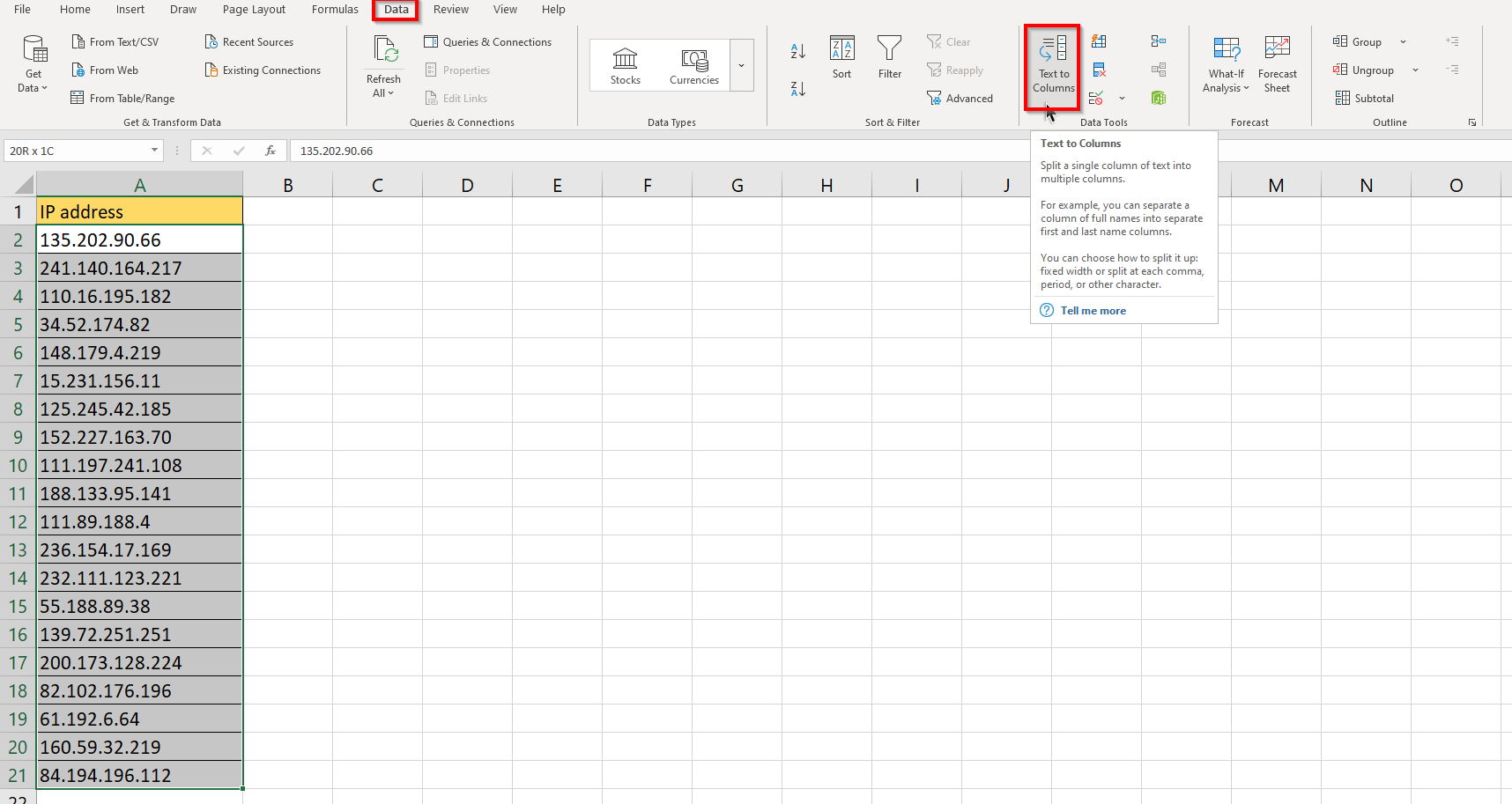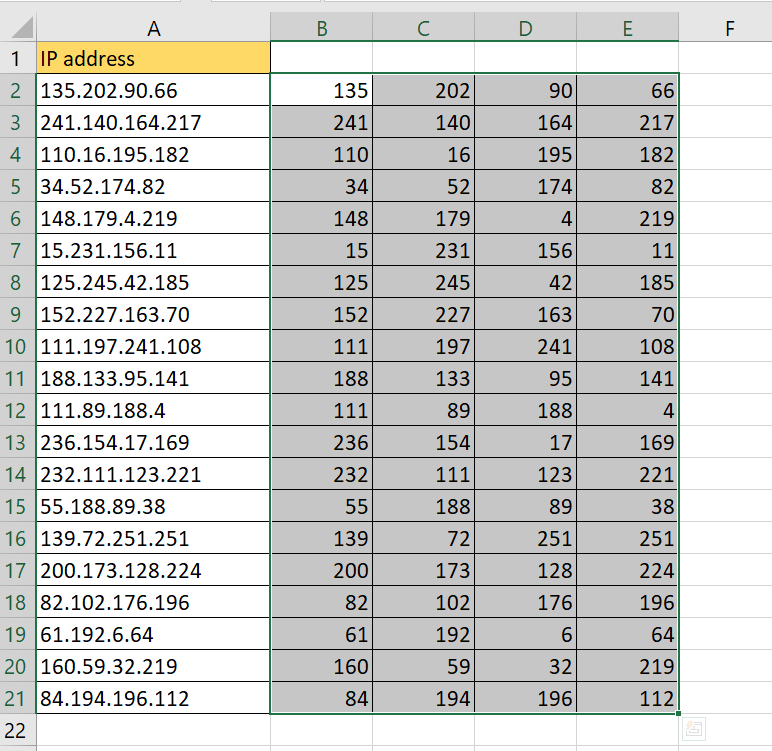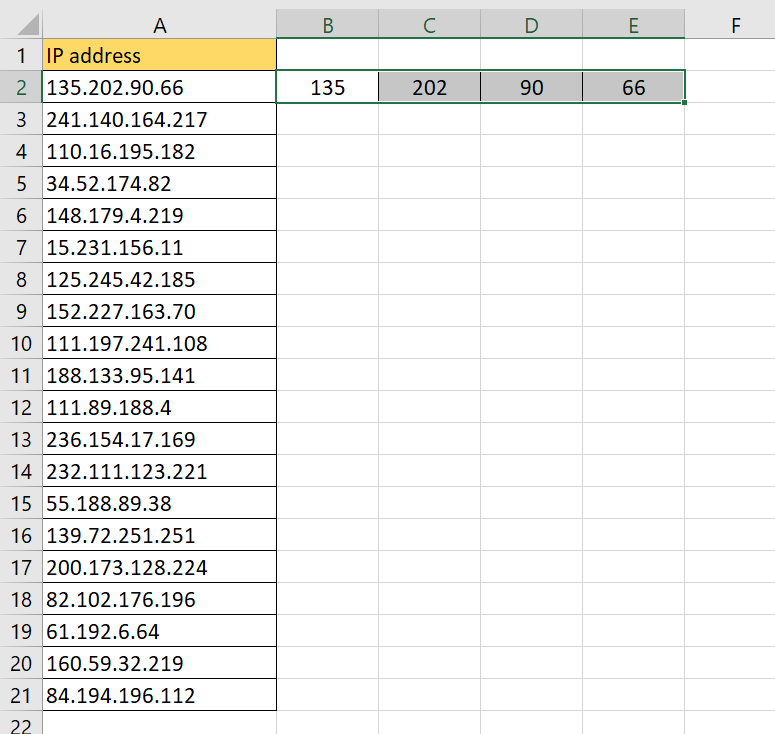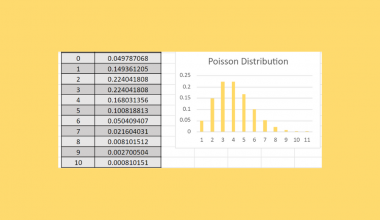# How to Extract a String from an IP Address in Excel

This guide will show how easy it is to extract a particular string from any IP address using tools and functions in Microsoft Excel.

IP addresses are 32-bit numbers that help identify the network interface on a machine. This number is represented using a dotted decimal format.

An IP Address is a unique address that is used to identify a device connected to the internet. The “IP” is short for Internet Protocol, referring to a set of rules that determine how to send data from one computer to another on the internet.

IP addresses look like a string of numbers with periods separating them into four sections. For example, the IP address used by most routers and modems is 192.168.0.1.

IPv4 addresses have a size of 32-bit. Each section can have a value ranging from 0 to 255, representing 8 bits. For example, the decimal value 192 is equivalent to “11000000” in binary.

The first three sections are the network ID. This part specifies the unique number and class assigned to the network. The last section is a unique identifier for the machine on your network known as the host ID.

Let’s say you want to only have the network ID or host ID from a list of IP addresses. How can we do this automatically in Microsoft Excel?

We can use the MID and FIND functions to return each 8-bit section of the IP address. After splitting the sections off, we can specify either the network ID or host ID in a separate column.

Alternatively, we can use the Text to Columns tool to use the periods as a delimiter. This tool allows us to convert delimited data into a table with multiple columns.

Let’s learn how to extract a string from an IP address ourselves in Excel and test the function with sample IPv4 addresses later.

## A Real Example of Extracting a String from an IP Address

Let’s look at an actual example of spreadsheets that try to extract strings from a given IP address.

In the table below, we have a list of IP addresses in column A. Columns B to E split the address into the different sections separated by periods.To get the values in Column C, we used the Text to Columns tool. This tool allows us to create new tables from delimited data. In this case, the “.” character is chosen as the delimiter, similar to how commas are usually used to delimit values in a CSV file.

If you want to extract a string from an IP address dynamically, we can use a formula that uses `MID` and `FIND` to look for specific sections of the IP address.

To get the string before the first dot, we use the following formula:

`=MID(A2,1,FIND(".",A2,1)-1)`

The `MID` function extracts a given number of characters from the middle of a certain text string. In this case, we want the section of the string starting from the first character to the position right before the first dot.

To get the position of the first dot, we use the `FIND` function. The `FIND` function returns the position of the first occurrence.

To find other substrings of an IP address, we must use nested `FIND` statements to get the particular sections of the IP address.

If you’re ready to extract a string from an IP address in Excel, let’s start writing it ourselves!

## How to Extract a String from an IP Address in Excel

This section will guide you through each step needed to start extracting a string from an IP address in Excel. You’ll learn how we can use the Text to Columns tool to split the IP address into separate sections quickly. We’ll also look at a more dynamic solution that uses the `MID` and `FIND` functions.

Follow these steps to start using the Text to Columns tool:

1. First, select the range of values that hold the IP addresses. In this example, we’ll select the range A2:A21.2. Next, we head over to the Data tab and look for the Text to Columns option. Click on the option to open the Convert Text to Columns Wizard dialog.3. In the first page of the Wizard, select the Delimited option and hit Next.4. When we get to the next page, choose Other as the delimiter and specify a dot or period ‘.‘ in the provided text box. Hit Next to proceed.5. For the final page, we can select a destination for our output columns. In this example, we can select cell B2 to be the start of our range.6. The Wizard should now have the IP address separated into different sections.If you want to create a dynamic solution, we can use the `MID` and `FIND` functions to create formulas to extract the different substrings of the IP address.

1. First, input the formulas in the ranges seen below to return each of the four sections. You can find a full list of the formulas in the sample spreadsheet shared earlier.2. Drag down the formulas to fill up the four columns. Since the table is dynamic, substituting any IP address here will result in new values in columns B to E.That’s all you need to remember to start extracting a string from an IP address in Excel. This step-by-step guide shows how easy it is to separate an IP address into individual 8-bit sections.

You can now use the `FIND` and `MID` functions in Excel and the various other Excel formulas available to create great worksheets that work best with you.

Are you interested in learning more about what Excel can do? Make sure to subscribe to our newsletter to be the first to know about the latest guides and tutorials from us.### Get emails from us about Excel.

Our goal this year is to create lots of rich, bite-sized tutorials for Excel users like you. If you liked this one, you'd love what we are working on! Readers receive ✨ early access ✨ to new content.

##### You May Also Like## How to Find and Replace Characters with a Superscript in Excel

The Find and Replace function can be used when you need to find and replace a character with…## How to Calculate Percentage Increase in Excel

This guide will discuss how to calculate percentage increase in Excel.  Calculating percentage increases on paper can be…## How to Plot Poisson Distribution in Excel

This guide will explain how to plot Poisson distribution in Excel using the POISSON.DIST function. Essentially, we first…## How To Use NPER Function in Excel

The NPER function in Excel is useful when you need to find out the number of periods for…